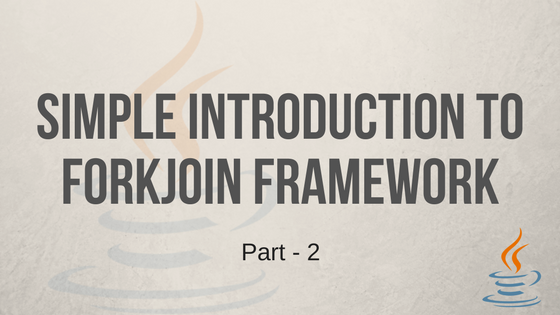Java developer, blogger and tech enthusiast.

# Simple Introduction to ForkJoin Framework - Part 2In the first part of this series we went through the introduction on ForkJoin framework. In this article we will go through code example to create a ForkJoinTask using RecursiveAction. RecursiveAction is an abstract class and it is one of the subclass of ForkJoinTask. In order to create a divisible task we need to extend RecursiveAction and implement compute() method.

In this article we will go through an array incrementor example. We declare a class ArrayIncrementor and this class will increment numbers in the given array and this class extends RecursiveAction. RecursiveAction has one abstract method, compute(), we implement this method. Instead of incrementing each element sequentially we will divide array into sub-arrays and threads in the pool will increment values in these sub-arrays. We need to take care of dividing array and ForkJoin framework will handle remaining things like execution of compute method and managing the threads.

To make it simple, lets start coding with small part of the class and then add code to it.

<1> Class ArrayIncrementor extends abstract RecursiveAction class. This class has abstract method compute() and we are going to implement it.
<2> This class increments values in the array so we declared integer array and also two integer fields ‘lo’ and ‘hi’ which contains lower and upper index limit of the array. Increments happens only with in this range. THRESHOLD is static and we maintain minimum sub-array size in it.
<3> ArrayIncrementor constructor gets input array, lower and higher indexes of array.
<4> Implements compute() method. This method is not yet fully implemented. We need to increment elements in the array only if ‘lo’ and ‘hi’ index is lower or equal to THRESHOLD else divide the array to make it smaller. This means computation happens only when task is small enough, else task is divided further.

In above code we created basic shell of the class. It is taking integer array with lo and hi index as input, now lets implement compute() method.

<1> If index range is equal or lower than THRESHOLD then, iterate array between lo and hi index and increment values. Note that ‘lo’ is inclusive and ‘hi’ is exclusive in the for loop.
<2> Calculating the mid index, if index range is greater than THRESHOLD. mid index will help us to divide array into two parts.
<3> Fork input array into two parts by calling invokeAll. We are passing two ArrayIncrementor objects to it, first object takes index range of lo to mid and second object takes mid to hi. Each ArrayIncrementor object can be forked further, if range is not in THRESHOLD limit, this call works like recursive call.

We implemented compute(), now lets create ForkJoinPool and pass this task to it.

<1> call invoke on ForkJoinPool by passing ArrayIncrementor object with array, lo and hi range. invoke() takes ForkJoinTask and because our ArrayIncrementor extends it, so we can pass it directly. In the next line we called shutdown to stop ForkJoinPool and then printed array.

Below will show the output

<1> Input is having 20 elements and the THRESHOLD is 5 so array is forked until size reaches five.
<2> compute happens only for five element range. The order of the compute is not sequential like 0-5 then 5-10 because they are executed by threads in parallel so there will be no order in execution.
<3> join statement printed after invokeAll execution.
<4> Final output shows incremented array values.

I added system outs in the code to show the flow of execution. In the above example there are four compute statements means those four tasks are executed in parallel. Below image which I posted in part 1 will help more in understanding this example.## Conclusion

We implemented RecursiveAction to for and increment array values. This is a simple example of using ForkJoin framework.